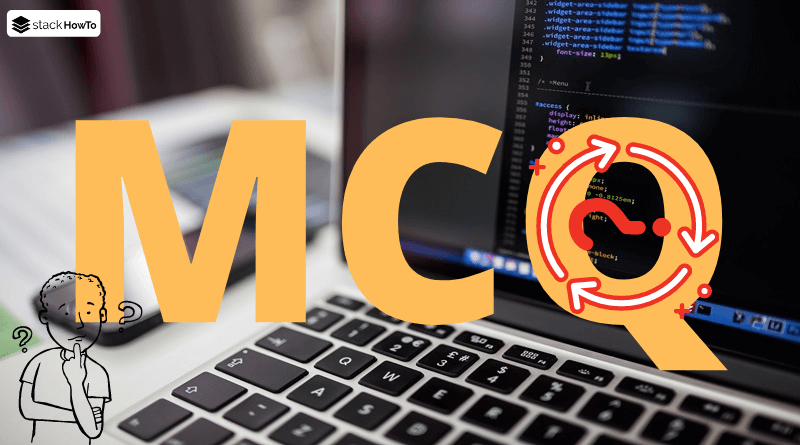MCQ

# Java MCQ – Multiple Choice Questions and Answers – Array – Part 2

This collection of Java Multiple Choice Questions and Answers (MCQs): Quizzes & Practice Tests with Answer focuses on “Java Array”.

###### 1. Which is the benefit of Java array?

A Size unlimited

B Random access

C Code Optimization

D None of the above

B, C
Random access and Code Optimization are the benefits of Java array.

###### 2. Array elements are stored in ________.

A Sequential memory

B Random memory

C Random & Sequential memory

D None of the above

A
In java, array elements are stored in sequential memory.

###### 3. What is the output of the following code?
```public class Q3
{
public static void main(String[] args)
{
int[] arr = new int;
System.out.print(arr.length);
}
}```

A Compilation error, the size of arrays can’t be initialized to zero.

B Compilation error, it’s arr.length() not arr.length

C 0

D None of the above

C
In java, size of arrays can be initialized with zero.

###### 4. What is the output of the following code?
```public class Q4
{
public static void main(String[] args)
{
int[] arr = {2, 3, 012 };

for(int i = 0; i < arr.length; i++)
System.out.print(arr[i] + " ");
}
}```

A 2 3 12

B 2 3 012

C 2 3 10

D 2 3 null

C
012 is an octal number. The prefix 0 shows that a number is in octal.

###### 5. Which keywords used to allocate memory to an array in Java?

A malloc

B allocate

C new

D construct

C
The keyword “new” allocates a block of memory determined by the size of an array, and provides the reference of memory allocated to the array.

###### 6. What is the output of the following code?
```int a[] = new int;
System.out.print(a);```

A Value stored in a

B 0

C 00000

C
The above code print the value stored in a.

###### 7. What is the output of the following code?
```public class Q7
{
public static void main(String args[])
{
int arr[] = new int;

for (int i = 0; i < 10; ++i)
{
arr[i] = i;
System.out.print(arr[i] + " ");
i++;
}
}
}```

A 0 2 4 6 8

B 1 3 5 7 9

C 0 1 2 3 4 5 6 7 8 9

D 1 2 3 4 5 6 7 8 9 10

A
Whenever an array is declared using the keyword “new” then all of its items are initialized to 0 automatically. “for” loop is executed 5 times as whenever controls enters the loop i value is incremented twice, first by i++ inside the loop then by ++i in increment condition of “for” loop.

###### 8. When you give an array to a method, the method receives ___________ of the array.

A The reference

B The length

C The first element

D A copy

A
When you give an array to a method, the method receives the reference of the array.

###### 9. What is the output of the following code?
```public class Q9
{
public static void main(String args[])
{
float[] arr = new float[]{1, 2, 3, 4};
System.out.println("Value is " + arr);
}
}```

A The program give a compile error due to the syntax `new float[]{1, 2, 3, 4}` is incorrect and it must be changed by `{1, 2, 3, 4}`.

B The code give a compile error due to the syntax `new float[]{1, 2, 3, 4}` is incorrect and it must be changed by `new float{1, 2, 3, 4};`

C The code give a compile error due to the syntax `new float[]{1, 2, 3, 4}` is incorrect and it must be changed by `new float[]{1.0, 2.0, 3.0, 4.0};`

D The program compiles with no errors.

D
The output : `Value is 2.0`

###### 10. What is the output of the following code?
```public class Q10
{
public static void main(String args[])
{
char arr [] = new char;

for (int i = 0; i < 5; ++i)
{
arr[i] = 'i';
System.out.print(arr[i] + "");
}
}
}```

A 1 2 3 4 5

B 1 2 3 4

C i j k l m

D i i i i i

D
The output is: `i i i i i`MCQPractice competitive and technical Multiple Choice Questions and Answers (MCQs) with simple and logical explanations to prepare for tests and interviews.Read More

### One thought on “Java MCQ – Multiple Choice Questions and Answers – Array – Part 2”

•maseczki na twarz

I’m extremely pleased to discover this website. I wanted to thank you for ones time just for this fantastic read!! I definitely enjoyed every part of it and i also have you bookmarked to see new stuff in your site.